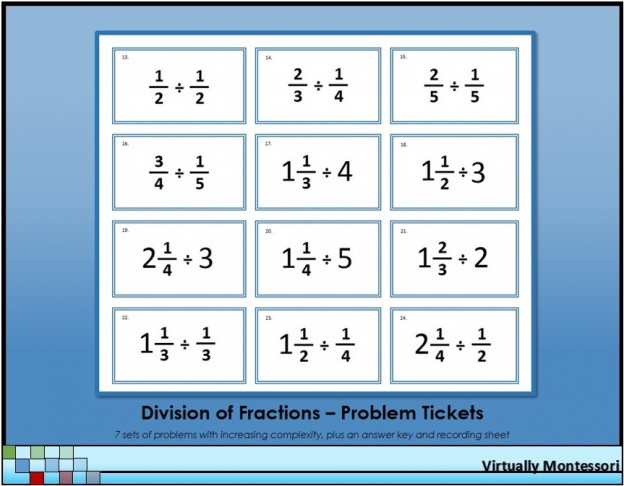# Division of Fractions Problem Tickets

These fraction problems are intended to be follow-up practice after a lesson. Each group of problems adds a new layer of complexity (see bulleted list below for how each problem set is differentiated). The blue border represents the division operation in Montessori math lessons and materials.
The following items are included in this material:

• Division of fractions problems tickets
• Problems #1-6: Division of a fraction by a whole number
• Problems #7-10: Division of a whole number by a fraction
• Problems #11-16: Division of a fraction by a fraction
• Problems #17-21: Division of a mixed number by a whole number
• Problems #22-26: Division of a mixed number by a fraction
• Problems #27-31: Division of a fraction by a mixed number
• Problems #32-36: Division of a mixed number by a mixed number